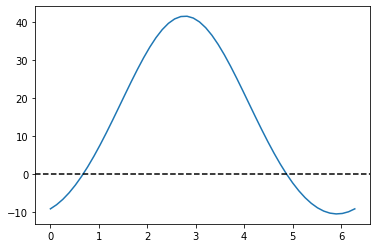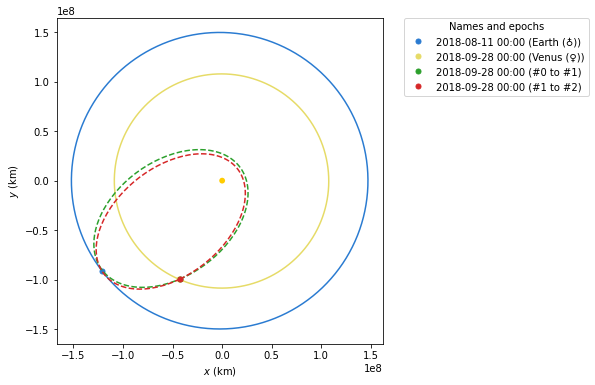# Analyzing the Parker Solar Probe flybys¶

## 1. Modulus of the exit velocity, some features of Orbit #2¶

First, using the data available in the reports, we try to compute some of the properties of orbit #2. This is not enough to completely define the trajectory, but will give us information later on in the process:

:

from astropy import units as u

:

T_ref = 150 * u.day
T_ref

:

$150 \; \mathrm{d}$
:

from poliastro.bodies import Earth, Sun, Venus

:

k = Sun.k
k

:

$1.3271244 \times 10^{20} \; \mathrm{\frac{m^{3}}{s^{2}}}$
:

import numpy as np

$T = 2 \pi \sqrt{\frac{a^3}{\mu}} \Rightarrow a = \sqrt{\frac{\mu T^2}{4 \pi^2}}$
:

a_ref = np.cbrt(k * T_ref**2 / (4 * np.pi**2)).to(u.km)
a_ref.to(u.au)

:

$0.55249526 \; \mathrm{AU}$
$\varepsilon = -\frac{\mu}{r} + \frac{v^2}{2} = -\frac{\mu}{2a} \Rightarrow v = +\sqrt{\frac{2\mu}{r} - \frac{\mu}{a}}$
:

energy_ref = (-k / (2 * a_ref)).to(u.J / u.kg)
energy_ref

:

$-8.0283755 \times 10^{8} \; \mathrm{\frac{J}{kg}}$
:

from astropy.time import Time

from poliastro.ephem import Ephem
from poliastro.util import norm

:

flyby_1_time = Time("2018-09-28", scale="tdb")
flyby_1_time

:

<Time object: scale='tdb' format='iso' value=2018-09-28 00:00:00.000>

:

r_mag_ref = norm(Ephem.from_body(Venus, flyby_1_time).rv().squeeze())
r_mag_ref.to(u.au)

:

$0.72573132 \; \mathrm{AU}$
:

v_mag_ref = np.sqrt(2 * k / r_mag_ref - k / a_ref)
v_mag_ref.to(u.km / u.s)

:

$28.967364 \; \mathrm{\frac{km}{s}}$

## 2. Lambert arc between #0 and #1¶

To compute the arrival velocity to Venus at flyby #1, we have the necessary data to solve the boundary value problem:

:

d_launch = Time("2018-08-11", scale="tdb")
d_launch

:

<Time object: scale='tdb' format='iso' value=2018-08-11 00:00:00.000>

:

r0, _ = Ephem.from_body(Earth, d_launch).rv()
r1, V = Ephem.from_body(Venus, flyby_1_time).rv()

:

r0 = r0
r1 = r1
V = V

:

tof = flyby_1_time - d_launch

:

from poliastro import iod

:

v0, v1_pre = iod.lambert(Sun.k, r0, r1, tof.to(u.s))

:

v0

:

$[9.5993373,~11.298552,~2.9244933] \; \mathrm{\frac{km}{s}}$
:

v1_pre

:

$[-16.980821,~23.307528,~9.1312908] \; \mathrm{\frac{km}{s}}$
:

norm(v1_pre)

:

$30.248465 \; \mathrm{\frac{km}{s}}$

## 3. Flyby #1 around Venus¶

We compute a flyby using poliastro with the default value of the entry angle, just to discover that the results do not match what we expected:

:

from poliastro.threebody.flybys import compute_flyby

:

V.to(u.km / u.day)

:

$[648499.74,~2695078.4,~1171563.7] \; \mathrm{\frac{km}{d}}$
:

h = 2548 * u.km

:

d_flyby_1 = Venus.R + h
d_flyby_1.to(u.km)

:

$8599.8 \; \mathrm{km}$
:

V_2_v_, delta_ = compute_flyby(v1_pre, V, Venus.k, d_flyby_1)

:

norm(V_2_v_)

:

$27.755339 \; \mathrm{\frac{km}{s}}$

## 4. Optimization¶

Now we will try to find the value of $$\theta$$ that satisfies our requirements:

:

from poliastro.twobody import Orbit

:

def func(theta):
V_2_v, _ = compute_flyby(v1_pre, V, Venus.k, d_flyby_1, theta * u.rad)
ss_1 = Orbit.from_vectors(Sun, r1, V_2_v, epoch=flyby_1_time)
return (ss_1.period - T_ref).to(u.day).value


There are two solutions:

:

from matplotlib import pyplot as plt

:

theta_range = np.linspace(0, 2 * np.pi)
plt.plot(theta_range, [func(theta) for theta in theta_range])
plt.axhline(0, color="k", linestyle="dashed")

:

<matplotlib.lines.Line2D at 0x7ff6100191f0>:

func(0)

:

-9.142672330001218

:

func(1)

:

7.098115439345711

:

from scipy.optimize import brentq

:

theta_opt_a = brentq(func, 0, 1) * u.rad
theta_opt_a.to(u.deg)

:

$38.598709 \; \mathrm{{}^{\circ}}$
:

theta_opt_b = brentq(func, 4, 5) * u.rad
theta_opt_b.to(u.deg)

:

$279.3477 \; \mathrm{{}^{\circ}}$
:

V_2_v_a, delta_a = compute_flyby(v1_pre, V, Venus.k, d_flyby_1, theta_opt_a)
V_2_v_b, delta_b = compute_flyby(v1_pre, V, Venus.k, d_flyby_1, theta_opt_b)

:

norm(V_2_v_a)

:

$29.978799 \; \mathrm{\frac{km}{s}}$
:

norm(V_2_v_b)

:

$29.491925 \; \mathrm{\frac{km}{s}}$

## 5. Exit orbit¶

And finally, we compute orbit #2 and check that the period is the expected one:

:

ss01 = Orbit.from_vectors(Sun, r1, v1_pre, epoch=flyby_1_time)
ss01

:

0 x 1 AU x 18.8 deg (HCRS) orbit around Sun (☉) at epoch 2018-09-28 00:00:00.000 (TDB)


The two solutions have different inclinations, so we still have to find out which is the good one. We can do this by computing the inclination over the ecliptic - however, as the original data was in the International Celestial Reference Frame (ICRF), whose fundamental plane is parallel to the Earth equator of a reference epoch, we have to change the plane to the Earth ecliptic, which is what the original reports use:

:

ss_1_a = Orbit.from_vectors(Sun, r1, V_2_v_a, epoch=flyby_1_time)
ss_1_a

:

0 x 1 AU x 24.0 deg (HCRS) orbit around Sun (☉) at epoch 2018-09-28 00:00:00.000 (TDB)

:

ss_1_b = Orbit.from_vectors(Sun, r1, V_2_v_b, epoch=flyby_1_time)
ss_1_b

:

0 x 1 AU x 13.3 deg (HCRS) orbit around Sun (☉) at epoch 2018-09-28 00:00:00.000 (TDB)

:

from poliastro.frames import Planes

:

ss_1_a.change_plane(Planes.EARTH_ECLIPTIC)

:

0 x 1 AU x 3.4 deg (HeliocentricEclipticIAU76) orbit around Sun (☉) at epoch 2018-09-28 00:00:00.000 (TDB)

:

ss_1_b.change_plane(Planes.EARTH_ECLIPTIC)

:

0 x 1 AU x 12.8 deg (HeliocentricEclipticIAU76) orbit around Sun (☉) at epoch 2018-09-28 00:00:00.000 (TDB)


Therefore, the correct option is the first one:

:

ss_1_a.period.to(u.day)

:

$158.75975 \; \mathrm{d}$
:

ss_1_a.a

:

$85839412 \; \mathrm{km}$

And, finally, we plot the solution:

:

from poliastro.plotting import StaticOrbitPlotter

frame = StaticOrbitPlotter(plane=Planes.EARTH_ECLIPTIC)

frame.plot_body_orbit(Earth, d_launch)
frame.plot_body_orbit(Venus, flyby_1_time)
frame.plot(ss01, label="#0 to #1", color="C2")
frame.plot(ss_1_a, label="#1 to #2", color="C3")

:

[<matplotlib.lines.Line2D at 0x7ff60e6cc670>,
<matplotlib.lines.Line2D at 0x7ff60e6cc9a0>]